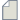Author Topic: Negative differential conductance in perfect zigzag GNR?  (Read 2082 times)

0 Members and 1 Guest are viewing this topic.pnprjt

• New ATK user
•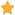• Posts: 4
• Country: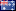• Reputation: 0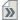Negative differential conductance in perfect zigzag GNR?
« on: July 1, 2013, 04:48 »
Hi all,

I have set up a simple device structure consisting of 8 zigzag GNRs (see attached image). I used the extended Huckel method with Cerda parameter set for Carbon and Hydrogen, performed the calculation self-consistently with energies = (-2,2,101), kpoints = (1,1,100). The calculation for bias from 0V to 0.6V in step 0.1V followed the tutorial http://quantumwise.com/publications/tutorials/mini-tutorials/98-i-v-curve-and-voltage-drop (see attached scripts).

The results show negative differential conductance in the I-V curve which is quite unexpected. Could you please let me know if there is anything miscalculated or any problem with the device set up?

Thank you very muchnori

• ATK Guru
•• Posts: 122
• Reputation: 12Re: Negative differential conductance in perfect zigzag GNR?
« Reply #1 on: July 1, 2013, 16:02 »
>if there is anything miscalculated or any problem with the device set up?
I think there is no problem in your calculation.

I recommend that you calculate the transmission eigenstate at fermi energy under finite bias both from left to right and  from right to left.pnprjt

• New ATK user
•• Posts: 4
• Country:• Reputation: 0Re: Negative differential conductance in perfect zigzag GNR?
« Reply #2 on: July 1, 2013, 19:15 »
Hi nori,

The result is unexpected for me because it contradicts with literature, e.g PRL 100, 206802 (2008). The I-V characteristics of perfect zigzag graphene ribbons should exhibit linear response. Here, the negative differential conductance appears even for small bias which is quite strange.nori

• ATK Guru
•• Posts: 122
• Reputation: 12Re: Negative differential conductance in perfect zigzag GNR?
« Reply #3 on: July 2, 2013, 01:53 »
>The I-V characteristics of perfect zigzag graphene ribbons should exhibit linear response.
This is the case only when N is odd in the literature and your structure is not odd but even.

I think what is importance is not negative differential conductance but that conductance is strongly reduced when N is even.Nordland

• QuantumATK Staff
• Supreme ATK Wizard
•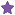• Posts: 812
• Reputation: 18Re: Negative differential conductance in perfect zigzag GNR?
« Reply #4 on: July 2, 2013, 09:02 »
When doing a simulation over a perfect system with biases, it is important to understand what it is the desired model.
If you apply a biases over such a system, it is no longer perfect, since the voltage drop introduces an asymmetry in the potential.
In a perfect system there is no natural place for the voltage drop to form and hence you get a slowed potential all the way through the scattering region,
which now will actually scatter electrons. This is of course correct if this is what you want to model is this.

If you want to model a perfect system with biases, you have to make infinite long when you apply the biases in order to avoid any scattering due to a potential slope,
but answer is in fact a much simpler one. The IV curve for a perfect system should always be calculated a zero biases calculation and then the IV curve should be calculated using linear response from the zero biases transmission spectrum. If you do this, it means that you would get the same results as in the literature.pnprjt

• New ATK user
•• Posts: 4
• Country:• Reputation: 0Re: Negative differential conductance in perfect zigzag GNR?
« Reply #5 on: July 2, 2013, 16:27 »
So, there is no problem with device set up or calculation. Then the result shows an interesting phenomenon for this type of structure.Nordland

• QuantumATK Staff
• Supreme ATK Wizard
•• Posts: 812
• Reputation: 18Re: Negative differential conductance in perfect zigzag GNR?
« Reply #6 on: July 2, 2013, 20:22 »
The true challenge is to map what you want model in the physical world to the proper simulation model.pnprjt

• New ATK user
•• Posts: 4
• Country:• Reputation: 0Re: Negative differential conductance in perfect zigzag GNR?
« Reply #7 on: July 3, 2013, 05:51 »
I have performed the self-consistent calculation for zigzag nanoribbons with N odd and the result indeed shows linear current voltage response with much higher conductance which agrees with literature.

Thanks